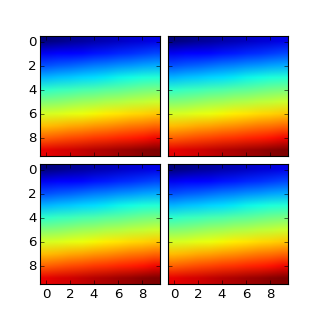### We're updating the default styles for Matplotlib 2.0

Learn what to expect in the new updates#### Previous topic

axes_grid example code: simple_anchored_artists.py

#### Next topic

axes_grid example code: simple_axesgrid2.py

# axes_grid example code: simple_axesgrid.py¶```import matplotlib.pyplot as plt
from mpl_toolkits.axes_grid1 import ImageGrid
import numpy as np

im = np.arange(100)
im.shape = 10, 10

fig = plt.figure(1, (4., 4.))
grid = ImageGrid(fig, 111, # similar to subplot(111)
nrows_ncols = (2, 2), # creates 2x2 grid of axes